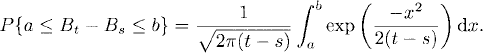# BROWNIAN MOTION

Brownian motion is a fundamentally important stochastic process in that it is a central notion throughout the theoretical development of stochastic processes. In this chapter, we present the definition and some properties of Brownian motion.

# 28.1 Basic Concepts and Facts

Definition 28.1 (Standard Brownian Motion). Let (Ω,, P) be a probability space. A stochastic process {Bt : t ≥ 0} on (Ω,, P) is called a standard Brownian motion if it satisfies the following conditions:

(a) P{B0 = 0} = P{ω : B0(ω) = 0} = 1.
(b) For any 0 ≤ s < t, the increment BtBs is a random variable normally distributed with mean 0 and variance ts, that is, for any a < b, we have(c) Bt has independent increments, that is, for any 0 ≤ t1 < t2 < … < tn, the random variables Bt1, Bt2Bt1,…, BtnBtn−1 are independent.
(d) Almost all sample paths of Bt are continuous functions:Definition 28.2 (Brownian Motion with Respect to Filtrations). Let {t : t ≥ 0} be a filtration. A stochastic ...

Get Measure, Probability, and Mathematical Finance: A Problem-Oriented Approach now with the O’Reilly learning platform.

O’Reilly members experience live online training, plus books, videos, and digital content from nearly 200 publishers.